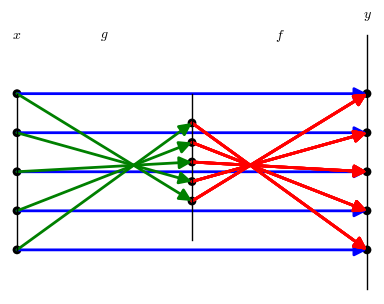Suppose $f$ and $g$ are functions, with domains $D_f$ and $D_g$, $f: D_f \rightarrow R$, and $g: D_g \rightarrow R$.
Definition:We say that $g$ is an inverse function for $f$ if (and only if)
(i) for every $x \in D_f, f(x) \in D_g$ , and $g(f(x))=x$, and
(ii) for every $x \in D_g, g(x) \in D_f$ , and $f(g(x))=x$.
Facts:
If $g$ is an inverse function for $f$ and $\hat g$ is an inverse function for $f$ then $g = \hat g$.
A function $f$ has an inverse function $g$ if and only if $f$ is one to one with $D_g = \{ y \in R : y= f(x)$ for some $x \in D_f\}$Diagram
INV.0 Mapping Diagram for $g$ as inverse of $f$ showing $f(g(x))=x$

Example
INV.REFL.0
Suppose $f(x) = -2x + 1$. Verify that $g(x) = -\frac {x-1} 2$ is the inverse function for $f$.